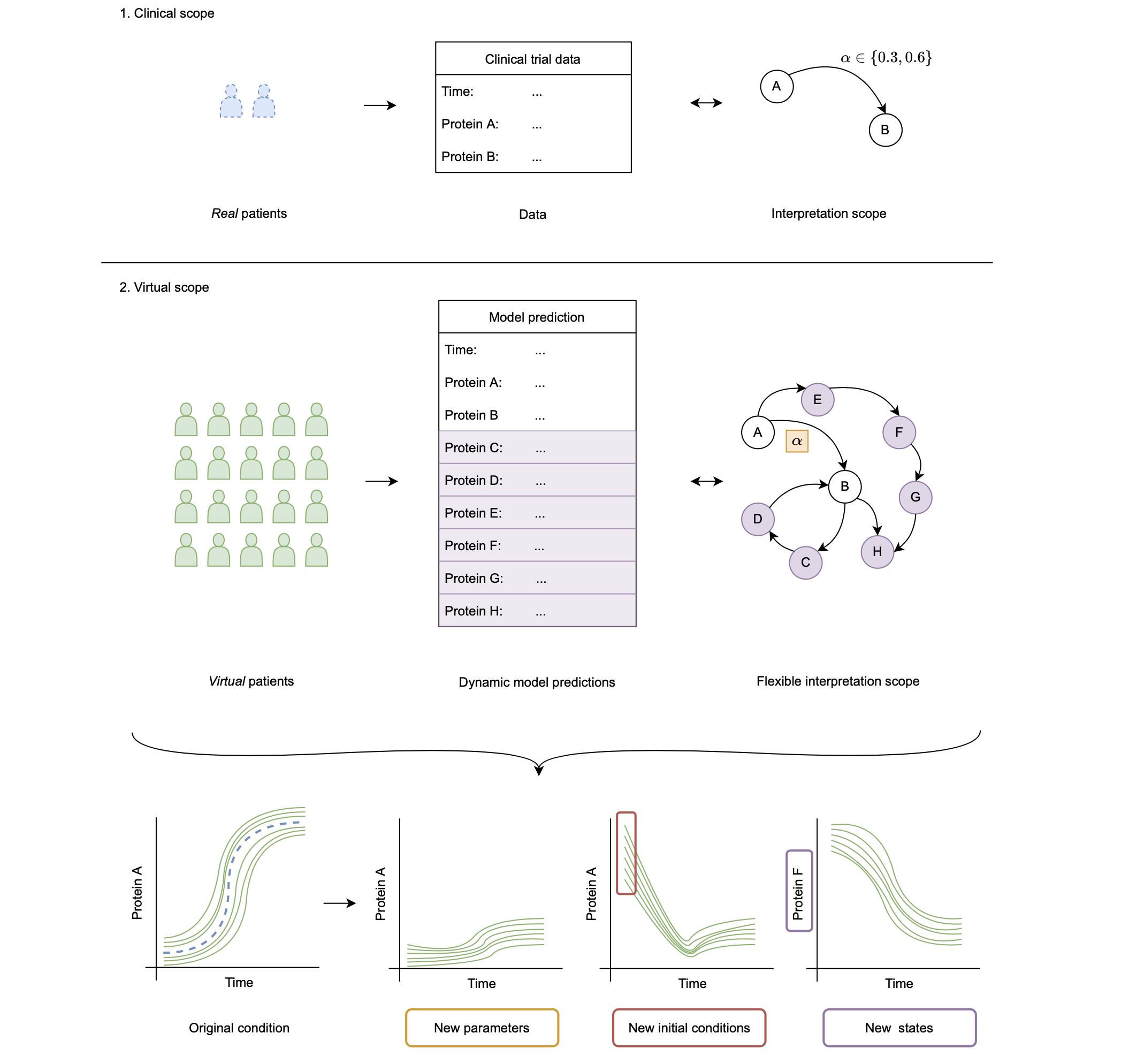# Parametric uncertainty quantification

In JuliaSimModelOptimizer one can perform parametric uncertainty quantification using parametric_uq with an InverseProblem. The result of a parametric_uq call is a set of parameters which sufficiently fit all experiments to their respective data simultaniously. We refer to this as the ensemble of plausible parameters, which can be subsequently subsampled following the subsampling API.

## The parametric_uq Function

JuliaSimModelOptimizer.parametric_uqFunction
parametric_uq(prob, alg; sample_size, adtype)

Create an ensemble of the given size of possible solutions for the inverse problem using the method specified by alg. This can be seen as a representation of the parametric uncertainty characterizing the given inverse problem. This is also called a multimodel in some domains.

Arguments

Keyword Arguments

• sample_size: Required. Number of samples to generate.
• adtype: Automatic differentiation choice, see the

Optimization.jl docs for details. Defaults to AutoForwardDiff().

Stochastic Optimization

If alg is a StochGlobalOpt method, the optimization algorithm runs until convergence or until maxiters has been reached. This process is repeated a number of times equal to sample_size to create an ensemble of possible parametrizations.

Markov Chain Monte Carlo (MCMC)

If alg is an MCMCOpt method, the ensemble is produced by drawing samples equal to sample_size from the posterior samples that the method has computed. This way, the variability in the ensemble solution depends on the uncertainty (variance) of the posterior distribution.

Note: It is recommended that maxiters > sample_size for MCMCOpt methods, so that the same parameter values are not sampled multiple times in the parametric_uq. Drawing a larger number of maxiters samples also helps to better characterize the variability around each parameter.

source

## Optimization Methods

The following are the choices of algorithms which are available for controlling the parametric uncertainty quantification process:

JuliaSimModelOptimizer.StochGlobalOptType
StochGlobalOpt(; method = SingleShooting(optimizer = BBO_adaptive_de_rand_1_bin_radiuslimited(),
maxiters = 10_000),
parallel_type=EnsembleSerial())

A stochastic global optimization method.

Keyword Arguments

• parallel_type: Choice of parallelism. Defaults to EnsembleSerial() or serial computation. For more information on ensemble parallelism types, see the ensemble types from SciML
• method: a calibration algorithm to use during the runs. Defaults to SingleShooting(optimizer=BBO_adaptive_de_rand_1_bin_radiuslimited(), maxiters=10_000). i.e. single shooting method with a black-box differential evolution method from BlackBoxOptimization. For more information on choosing optimizers, see Optimization.jl.
source
JuliaSimModelOptimizer.MCMCOptType
MCMCOpt(; maxiters, discard_initial = max(Int(round(maxiters/2)), 1000),
parallel_type = EnsembleSerial(), sampler = Turing.NUTS(0.65),
hierarchical = false)

A Markov-Chain Monte Carlo (MCMC) method for solving the inverse problem. The method approximates the posterior distribution of the model parameters given the data with a number of samples equal to maxiters.

Keyword Arguments

• maxiters: Required. Represents the number of posterior distribution samples.
• parallel_type: Choice of parallelism. Defaults to EnsembleSerial() or serial computation. Determines the number of chains of samples that will be drawn. For more information on ensemble parallelism types, see the ensemble types from SciML.
• discard_initial: The number of initial samples to discard. Defaults to the maximum between half of the maxiters samples and 1000, as a general recommendation. This default is the same as the number of samples that sampler draws during its warm-up phase, when the default NUTS sampler is used.
• sampler: The sampler used for the MCMC iterations. Defaults to Turing.NUTS for the No-U-Turn Sampler variant of Hamiltonian Monte Carlo. For more information on sampler algorithms, see the Turing.jl documentation
• hierarchical: Defaults to false. Controls whether hierarchical MCMC inference will be used. If it is set to true, each Trial object of the InverseProblem is treated as a separate subject and all subjects are assumed to come from the same population distribution. Population-level parameters are generated internally and their posterior is also inferred from data. If it is set to false, it is assumed that the same parameters are used for each subject and thus inference is performed on a lower-dimensional space.
source

## Result Types

JuliaSimModelOptimizer.ParameterEnsembleType
ParameterEnsemble

The structure contains the result of a parameter ensemble when a StochGlobalOpt method was used to generate the population. To export results to a DataFrame use DataFrame(ps) and to plot them use plot(ps, trial), where ps is the ParameterEnsemble and experiment is the AbstractExperiment, whose default parameter (or initial condition) values will be used.

source
JuliaSimModelOptimizer.MCMCResultType
MCMCResult

The structure contains the result of a plausible population when an MCMCOpt method was used to generate the population. To export results to a DataFrame use DataFrame(vp) and to plot them use plot(vp, trial), where vp is the VpopResult and trial is the AbstractExperiment, whose default parameter (or initial condition) values will be used.

The original Markov chain object that the MCMC method generated can be accessed using vp.original. This object contains summary statistics for each parameter and diagnostics of the MCMC method (e.g. Rhat and Effective Sample Size). See the MCMCChains.jl documentation for more information about diagnostic checks that can be run on vp.original.

source

## Importing Plausible Populations

If a plausible popualtion was generated in some alternative way, JuliaSimModelOptimizer still allows for importing these values into a VpopResult to enable using the virtual population analysis workflow. This is done via the following function:

Missing docstring.

Missing docstring for import_vpop. Check Documenter's build log for details.

## Analyzing Parameter Ensemble Results

Once VPs are generated given some multi-experiment data, we can use them to expand our analysis scope to dimensions where, for example, experimental data collection is difficult. This objective is schematically shown in the figure below. Here, we summarise three groups:

1. We can use the PS to model the system for new values of model parameters. Note that here we refer to model parameters not as parameters that specify a patient, but other, independent parameters of the model. As an example we have the reaction rate α.
2. We can use the PS to model the system for new initial conditions for the observed states of the sytem. Here, this relates to Protein A and B.
3. We can use the PS to model states of the system that have not been observed. These are Protein C to H in this example.The following functions enable such analyses: